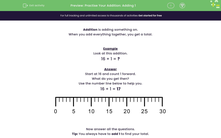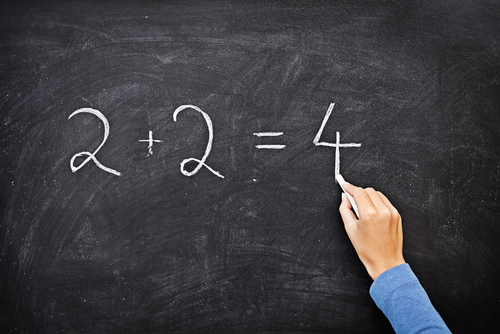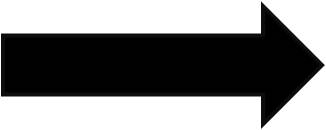### Comprehensive & curriculum aligned

In this worksheet, students will practise adding 1, 2 or 3 to a number to give a total (0-20).Key stage:  KS 1

Curriculum topic:   Number: Addition and Subtraction

Curriculum subtopic:   Add and Subtract to 20

Difficulty level:#### Worksheet Overview

When you add everything together, you get a total.Example

16 + 1 = ?

Start at 16 and count 1 forward.

What do you get then?

16 + 1 = 1722 + 2 = ?

This time, we start at 22 and count 2 forward. Again, you can use the number line to help you.

22 + 2 = 24

Always move to the right on a number line when you are adding.If we add on 3, then we need to count forward 3 numbers:

19 + 3 = 22

Have you got the idea?Let's try some questions now.

### What is EdPlace?

We're your National Curriculum aligned online education content provider helping each child succeed in English, maths and science from year 1 to GCSE. With an EdPlace account you’ll be able to track and measure progress, helping each child achieve their best. We build confidence and attainment by personalising each child’s learning at a level that suits them.

Get started